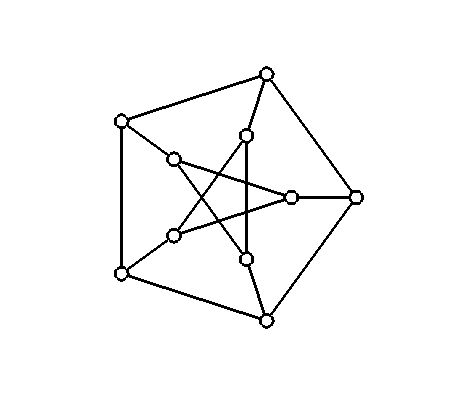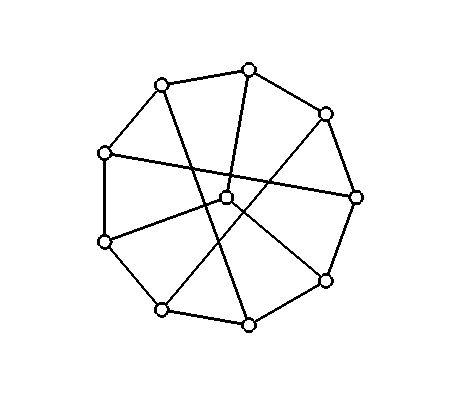## Homework 6 (due 10/30) CSC 491

We talked about finding the minimum spanning tree using the algorithms by Prim and Kruskal; we also saw the deceptively similar algorithm by Dijkstra for solving the single-source shortest path problem. Next week Dr. Rogers will start talking about the theory of NP-completeness.

Submission: you can submit the homework to me either by hardcopy in class or email it to me.

1. [Minimum Spanning Tree, 15pt] Do Exercise 23.2-8 (page 574) of the book about an alternative algorithm purporting to find a minimum spanning tree.

2. [Polynomial Time, 10pt] Do Exercise 34.104 (page 978) of the book, asking whether our dynamic programming solution for the 0/1 knapsack problem is a polynomial time solution.

3. [Graph Isomorphism, 10pt] Show that the following two graphs are isomorphic, i.e. drawings of the same graph, by naming the vertices of the two graphs so the vertices with the same name correspond to each other. Extra Credit: Can you find any other nice, regular drawings of this graph?4. [NP, 20pt] Do Exercise 34.2-1 (page 982) of the book showing that graph isomorphism is in NP.

5. [Finding a witness, 20pt] Do Exercise 34.2-3 of the book showing that if you can determine whether an arbitrary graph has a Hamiltonian cycle in polynomial time, then you can always find a Hamiltonian cycle in polynomial time.

6. [Extra Credit] Suppose you have a graph G =(V,E) and a minimum spanning tree T of G. The weight of one of the edges in G not belonging to T is lowered; can you find the minimum spanning tree of the new graph efficiently? Your running time should be O(|V|), that is, you don't have time to recompute the minimum spanning tree of G.

7. [Extra Credit] Show how to find a longest increasing subsequence of an array in time O(n log n).

8. [Extra Credit] Do Exercise 22.1-6 on finding a universal sink (a vertex with |V|-1 incomding edges, and no outgoing edges). It is rather tricky to get this to work in time O(|V|), and it will require cleverly combining some datastructures (like doubly linked lists).

Marcus Schaefer
Last updated: October 20th, 2006.